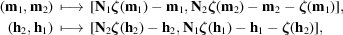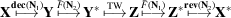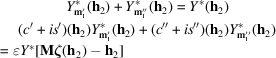International
Tables for
Crystallography
Volume B
Reciprocal space
Edited by U. Shmueli

International Tables for Crystallography (2010). Vol. B, ch. 1.3, pp. 87-88   | 1 | 2 |

Section 1.3.4.3.5.3. Complex symmetric and antisymmetric transforms

G. Bricognea

aGlobal Phasing Ltd, Sheraton House, Suites 14–16, Castle Park, Cambridge CB3 0AX, England, and LURE, Bâtiment 209D, Université Paris-Sud, 91405 Orsay, France

1.3.4.3.5.3. Complex symmetric and antisymmetric transforms

| top | pdf |

The matrixis its own contragredient, and hence (Section 1.3.2.4.2.2) the transform of a symmetric (respectively antisymmetric) function is symmetric (respectively antisymmetric). In this case the groupacts in both real and reciprocal space as. Ifwith both factors diagonal, thenacts byi.e.The symmetry or antisymmetry properties of X may be writtenwithfor symmetry andfor antisymmetry.

The computation will be summarized aswith the same indexing as that used for structure-factor calculation. In both cases it will be shown that a transformwithand M diagonal can be computed using onlypartial transformsinstead of.

 (i) Decimation in timeSincewe haveandmod, so that the symmetry relations for each parity class of datareador equivalentlyTransforming by, this relation becomesEach parity class thus obeys a different symmetry relation, so that we may multiplex them in pairs by forming for each pairthe vectorPuttingwe then have the demultiplexing relations for each:which can be solved recursively. Transform values at the exceptional pointswhere demultiplexing fails (i.e. where) can be accumulated while forming Y. Only the unique half of the values ofneed to be considered at the demultiplexing stage and at the subsequent TW and F(2I) stages. (ii) Decimation in frequencyThe vectors of final resultsfor each parity classobey the symmetry relationswhich are different for each. The vectorsof intermediate results after the twiddle-factor stage may then be multiplexed in pairs asAfter transforming by, the resultsmay be demultiplexed by using the relationswhich can be solved recursively as in Section 1.3.4.3.5.1(b)(ii).